Courses

# RD Sharma Solutions Ex-3.1, Rationalisation, Class 9, Maths Class 9 Notes | EduRev

## Class 9 : RD Sharma Solutions Ex-3.1, Rationalisation, Class 9, Maths Class 9 Notes | EduRev

The document RD Sharma Solutions Ex-3.1, Rationalisation, Class 9, Maths Class 9 Notes | EduRev is a part of the Class 9 Course RD Sharma Solutions for Class 9 Mathematics.
All you need of Class 9 at this link: Class 9

Q. 1. Simplify each of the following: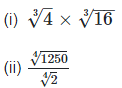Sol: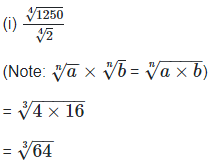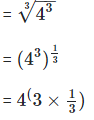= 41

= 4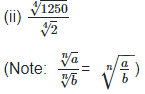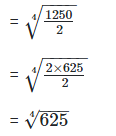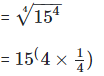= 15

Q. 2. Simplify the following expressions: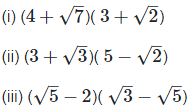Solution: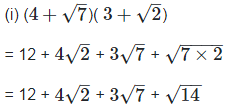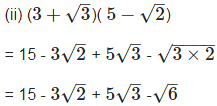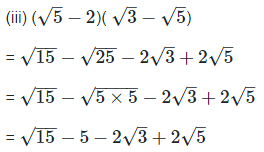Q. 3. Simplify the following expressions: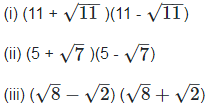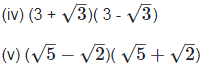Solution: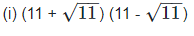As we know, (a + b) (a - b) = (a2−b2)

So, 112 – 11

121 –11 = 110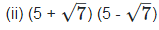As we know, (a + b) (a - b) = (a2−b2)

So, 52 – 7

25 – 7 = 18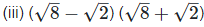As we know, (a + b) (a - b) = (a2−b2)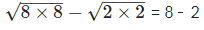= 6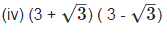As we know, (a + b) (a - b) = (a2−b2)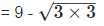= 6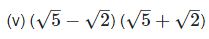As we know, (a + b) (a - b) = (a2−b2)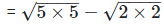= 5 – 2

= 3

Q. 4. Simplify the following expressions:Solution: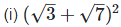As we know ,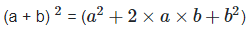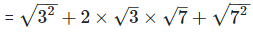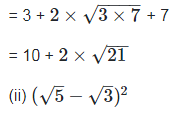As we know, (a - b)2 = (a2−2×a×b+b2)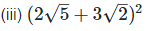As we know, (a + b)2 = (a2+2×a×b+b2)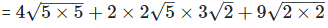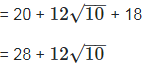Offer running on EduRev: Apply code STAYHOME200 to get INR 200 off on our premium plan EduRev Infinity!

91 docs

,

,

,

,

,

,

,

,

,

,

,

,

,

,

,

,

,

,

,

,

,

,

,

,

,

,

,

,

,

,

;